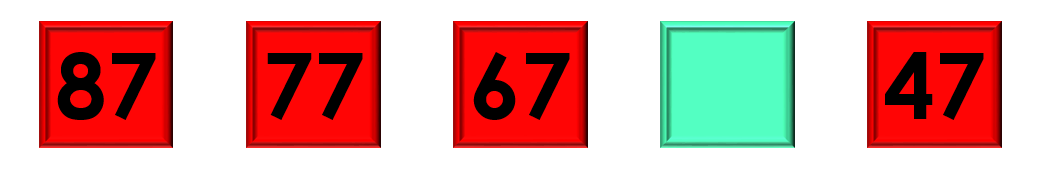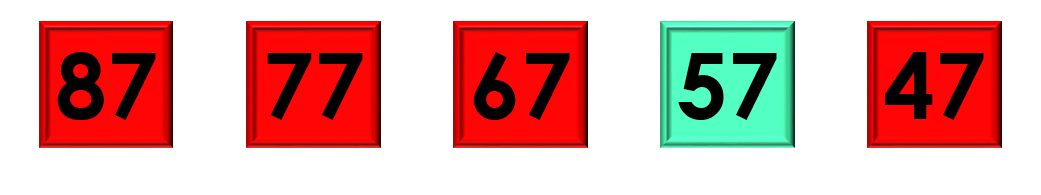1
visibility

Consider the given number pattern and identify the missing number.• A

57

• B

67

• C

55

• D

68

The correct answer is 57.

Solution:

Considering the given number pattern.Here, the numbers are decreasing.
So, to find the missing number, we first find the rule.

Note that:
87 – 10 = 77
77 – 10 = 67
67 – 10 = 57
57 – 10 = 47

So, the rule is "subtract 10".Then, the missing number is 57.